# Scaffold Q+S Kernel Density Estimation

The following document describes the kernel density function used in Scaffold Q+S.

Procedure

The basic data analyzed in Scaffold Q+S are intensities which, after applying a logarithmic transformation are generally roughly normally distributed within each sample. Suppose you have log-intensities {8,9,11,12,14} from protein P in Samples S.Figure 1. Intensity values

These values are taken from spectra. Since we are looking for differential expression and significance of protein expression, the first natural question is how to "roll up" these spectral values to get a protein-level value Vp.

The first suggestion might be to average them. We could use the mean, add the numbers and divide by 5 to get Vp = 10.8. Or, we could take the median, take the middle of the sorted numbers and get Vp = 11. But, we can do better by considering confidence and precision.

Suppose we have a level of trust for each value: say you trust the values at confidence 10%, 50%, 80%, 90% and 75% respectively.Figure 2. Intensity values and their confidence

Then you could do a weighted mean.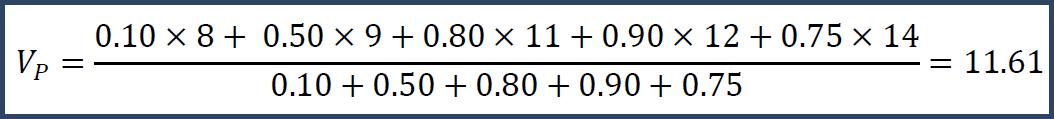Note that the weighted mean is larger than the unweighted mean because we have more confidence in the larger numbers. Or, we could do a weighted median and get Vp = 12. This calculation is more complicated, but Vp = 12 because that is the number where we can divide its trust so that the total confidence before it equals the total confidence after it.

Finally, suppose we had a notion of how precise each number was. We could replace each number with a Gaussian distribution centered at its value and standard deviation determined by the precision.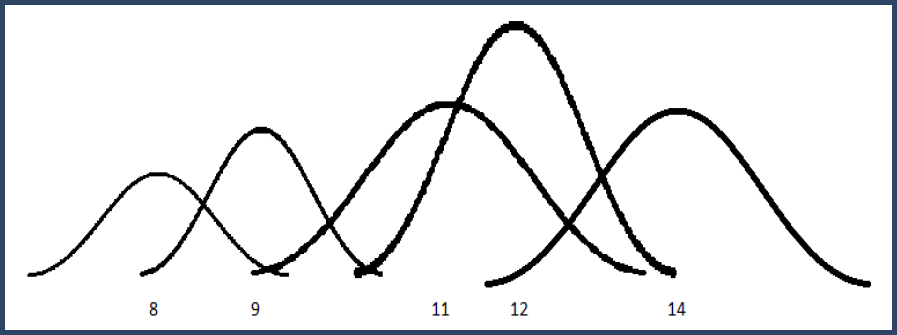Figure 3. Gaussian distributions for intensity values

This builds a mixture distribution where we combine the Gaussian "bumps" weighted by their level of confidence. The width of each Gaussian is determined by the precision and then the height is chosen so that the total area of the bump is equal to its confidence (weight).

The mixture distribution is the sum of these weighted Gaussian bumps. The median of this distribution is the point, shown below in green, where 50% of the area is to the left of the line and 50% is to the right. The mean is unaffected by these standard deviations; it is equal to the weighted mean.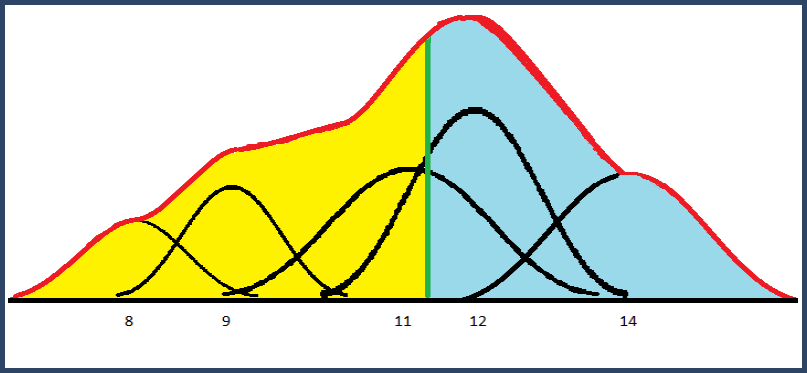Figure 4. Mixture distribution from summed Gaussian distributions

In the Proteins View, you can view the mixture distribution for the protein across the different categories and also its mean/median density distribution, which is the mixture distribution that comes from re-sampling the original mixture distributions with samples of size n (where n is the number of data points after rolling up to the current level of statistical blocking).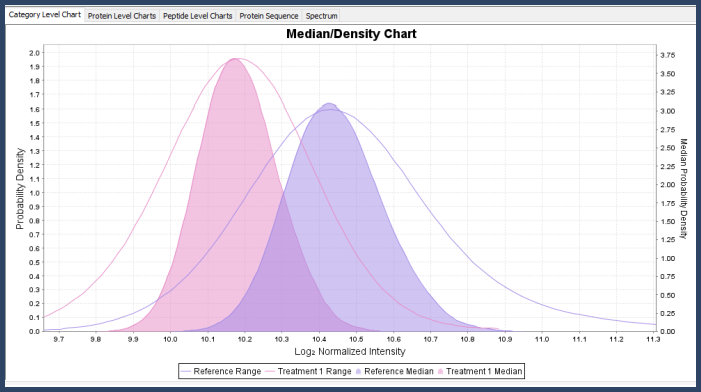Figure 5. Kernel density graph in Scaffold Q+S

Intensity Weighting and Standard Deviation Estimation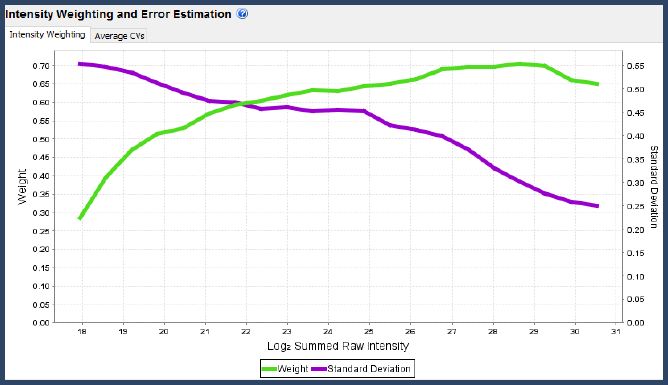Figure 6. Intensity weighting and error estimation graph in the Scaffold Q+S Statistics View

The confidence levels (weights) and standard deviations used by Scaffold Q+S are a function of the total summed raw log-intensity of each spectrum. The curved used can be viewed in the Statistics View. But, how does Scaffold determine these curves?

iTRAQ, TMT and SILAC

For iTRAQ, TMT and SILAC data, the curves are generated by first binning all spectra in the experiment into raw log-intensity bins (each bin having 100 spectra on average). Within each bin, and every multiplex channel, we calculate the set of differences between each spectrum's normalized value and the overall average in that channel. These differences are roughly normally distributed, so we can calculate a p-value for each value and from these, build a Bayesian probability histogram that measures the likelihood that points are scattered based on which bin their raw intensity falls into. This histogram is then smoothed and used as the weighting function. The standard deviation curve comes from taking the standard deviations of the differences in each bin and smoothing.

Generally these data-dependent curves should look as in the picture, with the green weighting function starting low and increasing to a peak among the highest raw intensities, then falling off a bit at the highest level of saturation.

Label-free

For label-free (precursor intensity) data, the above technique will not work due to the lack of multiplexes at the spectrum level. Instead we use a fixed curve.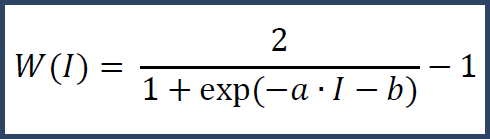I denotes the raw intensity and a and b are constants chosen to properly scale based on the distribution of raw intensities in the experiment. This curve places higher confidence in values with greater raw intensity. From this weighting curve we derive a matching theoretical standard deviation curve.

Intermediate Peptide Roll-up

In all cases, before being applied, the weights determined by intensity weighting are divided by the number of spectra matching each peptide. This adjustment allows for protein-level values to be seen as a roll-up of peptide-level values as opposed to a direct roll-up of spectrum values.